#### 期刊菜单

Gorenstein (m, n)-投射模
Gorenstein (m, n)-Projective Modules
DOI: 10.12677/PM.2020.1010118, PDF, HTML, XML, 下载: 410  浏览: 3,550  国家自然科学基金支持

Abstract: In this article, the notion of Gorenstein (m, n)-projective modules is introduced. Some properties of such modules are investigated over strongly left (m, n)-coherent rings, and some equivalent characterizations of Gorenstein (m, n)-projective modules are given.

1. 引言

2001年，作为n-内射模，P-内射模，FP-内射模的统一推广，Chen，Ding，Li和Zhou在文献  中引入了(m, n)-内射模的概念。2005年，Zhang等人在文献  中证明了在(m, n)-凝聚环上，(m, n)-内射左R-模的示性模是(m, n)-平坦的。同年，在文献  中，Mao和Ding在凝聚环上研究了模的FP-投射维数以及环的FP-投射整体维数。2006年，Mao和Ding在文献  中证明了 $\left({P}_{m,n},{I}_{m,n}\right)$ 是完备的余挠理论，其中 ${P}_{m,n}$ 是(m, n)-投射模类， ${I}_{m,n}$ 是(m, n)-内射模类。2014年，曾月迪在文献  中引入强(m, n)-凝聚环的概念，并证明了在强(m, n)-凝聚环上， $\left({P}_{m,n},{I}_{m,n}\right)$ 是完备遗传余挠对。2020年，杨强和赵仁育在文献  中研究了Gorenstein (m, n)-内射模，并在强(m, n)-凝聚环上利用Gorenstein (m, n)-内射模给出了左(m, n)-内射环的一些等价刻画。

2. 预备知识

mn是任取的两个正整数，R是具有单位元的结合环，所涉及的模均为左R-模。用 ${\text{id}}_{R}\left(M\right)$ 表示模M的内射维数，用 $\stackrel{˜}{I}$ 表示所有内射维数有限的左R-模类，对于左R-模M，用 ${M}^{+}={\text{Hom}}_{Z}\left(M,Q/Z\right)$ 表示M的示性模。

(2) (m, n)-投射模关于直和，直和项和扩张封闭。

3. Gorenstein (m, n)-投射模及其性质

$\Im =\cdots \to {P}_{1}\to {P}_{0}\to {P}_{-1}\to {P}_{-2}\to \cdots$,

Gorenstein (m, n)-投射左R-模的类记为 $G{P}_{m,n}$

(2) 若M是Gorenstein (m, n)-投射左R-模，则对任意 $E\in \stackrel{˜}{I}\cap {I}_{m,n}$${\text{Ext}}_{R}^{1}\left(M,E\right)=0$

(3) 若 $\Im =\cdots \to {P}_{1}\to {P}_{0}\to {P}_{-1}\to {P}_{-2}\to \cdots$ 是(m, n)-投射左R-模的正合序列，并对任意的 $E\in \stackrel{˜}{I}\cap {I}_{m,n}$${\text{Hom}}_{R}\left(\Im ,E\right)$ 正合，则每个箭头的像，核，余核都是Gorenstein (m, n)-投射左R-模；

(4) Gorenstein (m, n)-投射左R-模关于直积封闭。

${\Im }^{i}=\cdots \to {P}_{1}^{i}\to {P}_{0}^{i}\to {P}_{-1}^{i}\to {P}_{-2}^{i}\to \cdots$

${\oplus }_{i\in \text{I}}{\Im }^{i}=\cdots \to {\oplus }_{i\in \text{I}}{P}_{1}^{i}\to {\oplus }_{i\in \text{I}}{P}_{0}^{i}\to {\oplus }_{i\in \text{I}}{P}_{-1}^{i}\to {\oplus }_{i\in \text{I}}{P}_{-2}^{i}\to \cdots$

(1) 若M是Gorenstein (m, n)-投射左R-模，则对任意的 $E\in \stackrel{˜}{I}\cap {I}_{m,n}$，任意的 $i\ge 1$${\text{Ext}}_{R}^{\text{1}}\left(M,E\right)=0$

(2) 对任意左R-模的正合序列 $0\to N\to {G}_{k-1}\to \cdots \to {G}_{1}\to {G}_{0}\to M\to 0$${G}_{i}\left(0\le i\le k-1\right)$ 是Gorenstein (m, n)-投射模，则对任意的 $E\in \stackrel{˜}{I}\cap {I}_{m,n}$，以及任意的 $i>0$${\text{Ext}}_{R}^{i}\left(N,E\right)\cong {\text{Ext}}_{R}^{k+i}\left(M,E\right)$

$0\to M\to {P}_{0}\to {P}_{1}\to \cdots \to {P}_{k-1}\to {P}_{0}\to N\to 0$

(2) 的证明由(1)可得。

$\Im =\cdots \to {P}_{1}\to {P}_{0}\to {P}_{-1}\to {P}_{-2}\to \cdots$

• )设N是(m, n)-内射左R-模，且 ${\text{id}}_{R}\left(N\right)=k<\infty$。下证 ${\text{Hom}}_{R}\left(\Im ,N\right)$ 正合，对k进行归纳总结。当 $k=0$ 时，结论显然成立。设 $k\ge 1$，考虑短正合序列

$0\to N\to E\to L\to 0$

$0\to {L}^{+}\to {E}^{+}\to {N}^{+}\to 0$

$0\to {\text{Hom}}_{R}\left(\Im ,N\right)\to {\text{Hom}}_{R}\left(\Im ,E\right)\to {\text{Hom}}_{R}\left(\Im ,L\right)\to 0$

(1) M是Gorenstein (m, n)-投射模；

(2) 存在左R-模的正合序列 $0\to M\to {P}_{-1}\to {P}_{-2}\to \cdots$，其中每个 ${P}_{i}$ 是(m, n)-投射模；

(3) 存在左R-模的短正合序列 $0\to M\to P\to L\to 0$，其中P是(m, n)-投射模，L是Gorenstein (m, n)-投射模。

(2) Þ (1)设 $\cdots \to {P}_{1}\to {P}_{0}\to M\to 0$ 是(m, n)-投射分解。由(2)知，存在左R-模的正合序列

$0\to M\to {P}_{-1}\to {P}_{-2}\to \cdots$

$\cdots \to {P}_{1}\to {P}_{0}\to {P}_{-1}\to {P}_{-2}\to \cdots$

(1) 若 ${M}_{1}$ 是Gorenstein (m, n)-投射模， ${M}_{3}$ 是(m, n)-投射模，则 ${M}_{2}$ 是Gorenstein (m, n)-投射模；

(2) 若 ${M}_{3}$ 是Gorenstein (m, n)-投射模， ${M}_{2}$ 是(m, n)-投射模，则 ${M}_{1}$ 是Gorenstein (m, n)-投射模。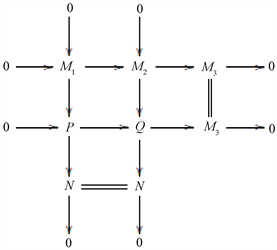Figure 1. Pushout diagram

(2) 类似地，由推论3.6可得。

(1) 每个左R-模是Gorenstein (m, n)-投射的；

(2) 环R满足以下两个条件：

(i) 每个内射左R-模是(m, n)-投射的；

(ii) 每个内射维数有限的(m, n)-内射左R-模是内射的。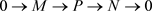(2) Þ (1) 设M是左R-模。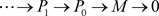M的一个(m, n)-投射分解，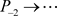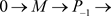M的一个内射分解，由(i)知存在(m, n)-投射左R-模的正合序列Auslander, M. and Bridger, M. (1969) Stable Module Theory. In: Memoirs of the American Mathematical Society, 94. https://doi.org/10.1090/memo/0094  Enoch, E.E. and Jenda, O.M.G. (1995) Gorenstein Injective and Projective Modules. Mathematische Zeitschrift 220, 611-633. https://doi.org/10.1007/BF02572634  Christensen, L.W. (2000) Gorenstein Dimension. In: Lecture Notes in Math, Springer-Verlag, Berlin. https://doi.org/10.1007/BFb0103984  Enoch, E.E. and Jenda, O.M.G. (2000) Relative Homological Algerbra. Walter de Gruyter, Berlin. https://doi.org/10.1515/9783110803662  Holm, H. (2004) Gorenstein Homological Dimensions. Journal of Pure and Applied Algebra, 189, 167-193. https://doi.org/10.1016/j.jpaa.2003.11.007  Holm, H. (2004) Gorenstein Derived Functors. Proceedings of the American Mathematical Society, 132, 1913-1923. https://doi.org/10.1090/S0002-9939-04-07317-4  Chen, J.L., Ding, N.Q., Li, Y.L. and Zhou, Y.Q. (2001) On (m, n)-Injectivity of Modules. Communications in Algebra, 29, 5589-5603. https://doi.org/10.1081/AGB-100107948  Zhang, X.X., Chen, J.L. and Zhang, J. (2005) On (m, n)-Injective Modules and (m, n)-Coherent Rings. Algebra Colloquium, 12, 149-160. https://doi.org/10.1142/S1005386705000143  Mao, L.X. and Ding, N.Q. (2005) Fp-Projective Dimensions. Communications in Algebra, 33, 1153-1170. https://doi.org/10.1081/AGB-200053832  Mao, L.X. and Ding, N.Q. (2006) On Relative Injective Modules and Relative Coherent Rings. Communications in Algebra, 34, 2531-2545. https://doi.org/10.1080/00927870600651208  曾月迪. 强(m, n)-凝聚环[J]. 江南大学学报(自然科学版), 2014, 13(5): 611-615.  杨强, 赵仁育. Gorenstein (m, n)-内射模[J]. 山东大学学报(理学版), 2020, 55(12): 18-22.  Rotman, J.J. (1979) An Introduction to Homological Algebra. Academic Press, New York.